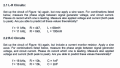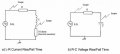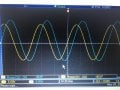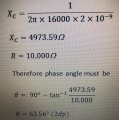Question regarding phase angle between inductor current and supply voltage

Lavanoj

Joined Nov 10, 2017
7
Hi,

To measure the phase angle between the inductor current and supply voltage we used an osciliscope and measured the angle between them. We plugged an osciliscope into the circuit measuring the voltage across a 10ohm resistor and called this a "current monitor". To work out the phase angle between the when I used a 68mH inductor and a 4.7kohm resistor the phase angle was 45 degrees.

The next step was to prove this with theory.

The formula is: tan^-1(Xl/R) I get that because you are doing the impedance triangle. This gives a phase angle of 45 degrees. However, how is this measuring the phase angle between the inductor CURRENT and voltage SUPPY. I would have thought this is giving the phase angle between inductor VOLTAGE and voltage supply not the current. Unless I am misunderstanding some theory that you may be able to explain.

WBahn

Joined Mar 31, 2012
26,398
What is the relationship between the phase angle of the current in the monitoring resistor and the inductor?

What is the relationship between the phase angle of the voltage in the monitoring resistor and the current through it?

Lavanoj

Joined Nov 10, 2017
7
What is the relationship between the phase angle of the current in the monitoring resistor and the inductor?

What is the relationship between the phase angle of the voltage in the monitoring resistor and the current through it?
1. I would have thought the phase angles would be zero, due to the current in the circuit being the same as they’re in series.

2. I would have thought the voltage would be in phase again due to the inductor being before the current monitor resistor

WBahn

Joined Mar 31, 2012
26,398
1. I would have thought the phase angles would be zero, due to the current in the circuit being the same as they’re in series.
Correct. The phase angle for the current through components in series are the same. Similarly, the phase angle for the voltage across components in parallel are the same.

2. I would have thought the voltage would be in phase again due to the inductor being before the current monitor resistor
Has absolutely nothing to do with where the inductor is or whether there even is an inductor.

The current through and voltage across a resistor are in phase. Period.

Similarly, the voltage across an inductor leads the current through an inductor by 90° while the voltage across a capacitor lags the current through a capacitor by 90°.

Of course, we are talking about sinusoidal signals here, but it applies to each frequency component even of non-sinusoidal components.

Have you been introduced to complex impedance?

Lavanoj

Joined Nov 10, 2017
7

Would I be correct in saying this....

I have been asked to measure the phase angle beteeen the inductor current and supply voltage. If we look at the voltage in the current monitor, it will be in phase with the inductor current. Therefore, the phase angle between the current monitor resistor voltage and supply voltage will be the same as the angle between the inductor and the supply voltage.

In this circumstance I used the scope and got an angle of 90 - tan-1(Xc/R). Took me a while to realise the 90 - bit through trial and error. Can you explain why I have to do 90 - in this case? I was thinking it might be due to voltage and current being 90 degrees out of phase maybe? For the inductor questions I had 45 degrees for both answers so I am not sure if you are supposed to do 90 - for those cause I’d get the same answer.

I can send you the question if that would make what I have said easier to understand.

Yes I have been introduced to complex circuit imaginary numbers etc.

WBahn

Joined Mar 31, 2012
26,398

Would I be correct in saying this....

I have been asked to measure the phase angle beteeen the inductor current and supply voltage. If we look at the voltage in the current monitor, it will be in phase with the inductor current. Therefore, the phase angle between the current monitor resistor voltage and supply voltage will be the same as the angle between the inductor and the supply voltage.
Yes. That is the idea.

In this circumstance I used the scope and got an angle of 90 - tan-1(Xc/R). Took me a while to realise the 90 - bit through trial and error. Can you explain why I have to do 90 - in this case? I was thinking it might be due to voltage and current being 90 degrees out of phase maybe? For the inductor questions I had 45 degrees for both answers so I am not sure if you are supposed to do 90 - for those cause I’d get the same answer.

I can send you the question if that would make what I have said easier to understand.

Yes I have been introduced to complex circuit imaginary numbers etc.
What would really help is to send not only the question that was asked, but also your work on it. That way we can see where you might be going wrong instead of mostly guessing.

Lavanoj

Joined Nov 10, 2017
7

I will attach the images to make it easier for you to see what I have done.Question 2.2 I have attached the graph and the maths below, I am unsure why for this one I need to do 90 minus before the calculation.In the scope image the blue line is the capacitor current/voltage (I think the scope only measures voltage) and the yellow line is the supply voltage. I am not sure whether I have done the measuring wrong or whether my calculations are wrong. You are correct in saying it is a guess at the moment.

I appreciate the help.

Lavanoj

Joined Nov 10, 2017
7
Yes. That is the idea.

What would really help is to send not only the question that was asked, but also your work on it. That way we can see where you might be going wrong instead of mostly guessing.